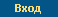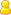Логин: Пароль: Забыли пароль?русский | englishВойти | Регистрация | Помощь

# Семинар Добрушинской математической лаборатории ИППИ РАН

Семинар Добрушинской математической лаборатории ИППИ РАН

29 декабря, вторник, 16:00 пройдет в Zoom по обычному адресу (см. ниже).

Vassili Kolokoltsov (Warwick Uni):
Continuous time random walks modeling of quantum measurement and
fractional equations of quantum stochastic filtering and control.

Abstract:
Initially developed in the framework of quantum stochastic calculus,
the main equations of quantum stochastic filtering were later on derived
as the limits of Markov models of discrete measurements under appropriate
scaling. In many branches of modern physics it became popular to extend
random walk modeling to the continuous time random walk (CTRW) modeling,
where the time between discrete events is taken to be non-exponential.
In the present work we apply the CTRW modeling to the continuous
quantum measurements yielding the new fractional in time evolution
equations of quantum filtering and thus new fractional equations of
quantum mechanics of open systems. The related quantum control problems
and games turn out to be described by the fractional Hamilton-Jacobi-Bellman
(HJB) equations on Riemannian manifolds. By-passing we provide a full
derivation of the standard quantum filtering equations, in a modified
way as compared with existing texts, which provides explicit rates of
convergence (that were not available via the tightness of martingales
approach developed previously). The talk will be based on the author"s
preprint https://arXiv:2008.07355

Ссылка для подключения:

https://zoom.us/j/93175142429?pwd=VDViRHNOSlZSVUM5ZU03SGZyZy8xQT09

Id: 931-7514-2429 passw=057376

Пожалуйста регистрируйте участие в Zoom по вашему имени и фамилии,
а не псевдонимом. Переименовать себя можно прямо во время сессии.

 23.12.2020 | Комеч Сергей Александрович

© Федеральное государственное бюджетное учреждение науки
Институт проблем передачи информации им. А.А. Харкевича Российской академии наук, 2021
Об институте  |  Контакты  |  Старая версия сайта